pandas对纯文本的读取提供了非常强力的支持，参数有四五十个。这些参数中，有的很容易被忽略，但是在实际工作中却用处很大。`pd.read_csv()` 的格式如下：

``````read_csv(
sep: str = ...,
delimiter: str | None = ...,
header: int | Sequence[int] | str = ...,
names: Sequence[str] | None = ...,
index_col: int | str | Sequence | Literal[False] | None = ...,
usecols: int | str | Sequence | None = ...,
squeeze: bool = ...,
prefix: str | None = ...,
mangle_dupe_cols: bool = ...,
dtype: str | Mapping[str, Any] | None = ...,
engine: str | None = ...,
converters: Mapping[int | str, (*args, **kwargs) -> Any] | None = ...,
true_values: Sequence[Scalar] | None = ...,
false_values: Sequence[Scalar] | None = ...,
skipinitialspace: bool = ...,
skiprows: Sequence | int | (*args, **kwargs) -> Any | None = ...,
skipfooter: int = ..., nrows: int | None = ..., na_values=...,
keep_default_na: bool = ..., na_filter: bool = ...,
verbose: bool = ..., skip_blank_lines: bool = ...,
parse_dates: bool | List[int] | List[str] = ...,
infer_datetime_format: bool = ...,
keep_date_col: bool = ...,
date_parser: (*args, **kwargs) -> Any | None = ...,
dayfirst: bool = ..., cache_dates: bool = ...,
iterator: Literal[True],
chunksize: int | None = ...,
compression: str | None = ...,
thousands: str | None = ...,
decimal: str | None = ...,
lineterminator: str | None = ...,
quotechar: str = ...,
quoting: int = ...,
doublequote: bool = ...,
escapechar: str | None = ...,
comment: str | None = ...,
encoding: str | None = ...,
dialect: str | None = ...,
delim_whitespace: bool = ...,
low_memory: bool = ...,
memory_map: bool = ...,
float_precision: str | None = ...)``````

## 1. FilePathOrBuffer

``````# 文件路径读取
file_path=r"E:\VSCODE\2_numpy_pandas\pandas\Game_Data.csv"
print(f_df)

# 网页上的文件读取

# 文件对象读取
f = open(r"E:\VSCODE\2_numpy_pandas\pandas\Game_Data.csv", encoding="gbk")

## 2. sep

``f_df = pd.read_csv(file_path,sep=":|;",engine="python",header=0)``

## 3. delim_whitespace（不常用）

``````file_path=r"E:\VSCODE\2_numpy_pandas\pandas\Game_Data.csv"
print(df)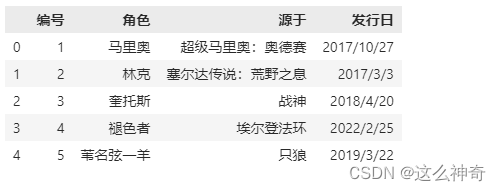``````pd.read_csv(file_path,sep=",|:|;",engine="python",header=1,encoding='gbk')``pd.read_csv(file_path,sep=",|:|;",engine="python",encoding='gbk',names=["编号", "英雄", "游戏", "发行日期"])````pd.read_csv(file_path,sep=",|:|;",engine="python",encoding='gbk',names=["编号", "英雄", "游戏", "发行日期"],header=0)``## 5. index_col

``pd.read_csv(file_path,engine="python",encoding='gbk',header=0,index_col="角色")``## 6. usecols

``pd.read_csv(file_path,encoding='gbk',usecols=["角色", "发行日"])``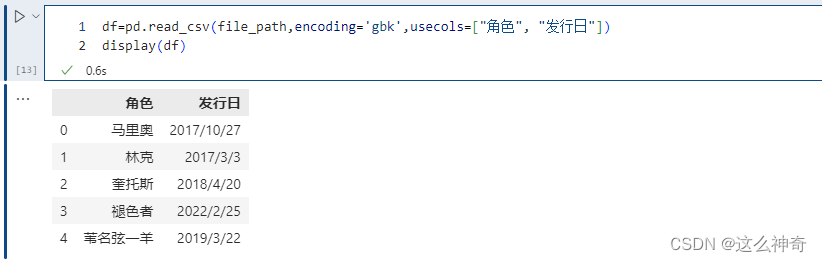``````# 选择列名的长度等于 3 的列，显然此时只会选择 发行日 这一列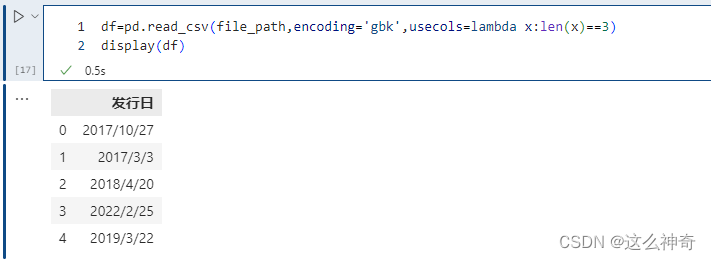## 7. mangle_dupe_cols

``# ValueError: Setting mangle_dupe_cols=False is not supported yet``

## 8. prefix

``pd.read_csv(file_path,encoding='gbk',header=None,prefix="角色")``## 9. dtype

``df=pd.read_csv(file_path,encoding='gbk',dtype={"编号": str})df["编号"]=df["编号"]*4``## 10. engine

pandas解析数据时用的引擎，pandas 目前的解析引擎提供两种：c、python，默认为 c，因为 c 引擎解析速度更快，但是特性没有 python 引擎全。如果使用 c 引擎没有的特性时，会自动退化为 python 引擎。

``````df=pd.read_csv(file_path,encoding='gbk',dtype={"编号": str})
df["编号"]=df["编号"]*4``````## 11. converters

``pd.read_csv(file_path,encoding='gbk', converters={<!--{cke_protected}{C}%3C!%2D%2D%20%2D%2D%3E-->"编号": lambda x: int(x) + 10})``## 12. true_values和false_value

``pd.read_csv(file_path,encoding='gbk',true_values=["林克","奎托斯","褪色者","苇名弦一羊"],false_values=["马里奥"])````pd.read_csv(file_path,encoding='gbk',true_values=["林克"],false_values=["马里奥"])``## 13. skiprows

skiprows 表示过滤行，想过滤掉哪些行，就写在一个列表里面传递给skiprows即可。注意的是：这里是先过滤，然后再确定表头，比如：

``pd.read_csv(file_path,encoding='gbk',skiprows=)``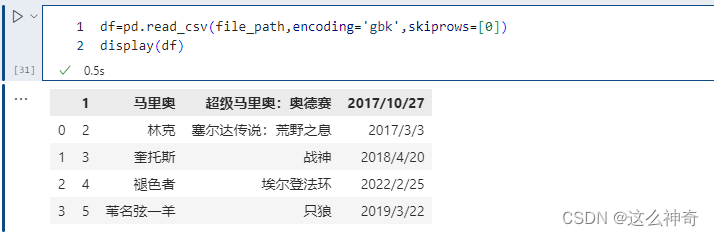``pd.read_csv(file_path,encoding='gbk',skiprows=lambda x:x>0 and x%2==1)``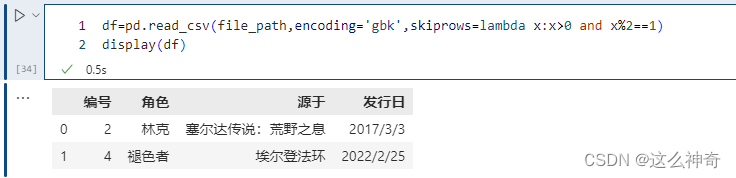## 14. skipfooter

``pd.read_csv(file_path,encoding='gbk',skipfooter=2)``skipfooter接收整型，表示从结尾往上过滤掉指定数量的行，因为引擎退化为python，那么要手动指定engine=“python”，不然会警告。

## 15. nrows

nrows 参数设置一次性读入的文件行数，它在读入大文件时很有用，比如 16G 内存的PC无法容纳几百 G 的大文件。

``pd.read_csv(file_path,encoding='gbk',nrows=4)``## 16. na_values

na_values 参数可以配置哪些值需要处理成 NaN，这个是非常常用的。

`pd.read_csv(file_path,encoding='gbk',na_values=['马里奥','战神'])```pd.read_csv(file_path,encoding='gbk',na_values={<!--{cke_protected}{C}%3C!%2D%2D%20%2D%2D%3E-->"角色":['马里奥','战神'],'编号':})``## 19. skip_blank_lines

skip_blank_lines 默认为 True，表示过滤掉空行，如为 False 则解析为 NaN。

## 21. date_parser

``````from datetime import datetime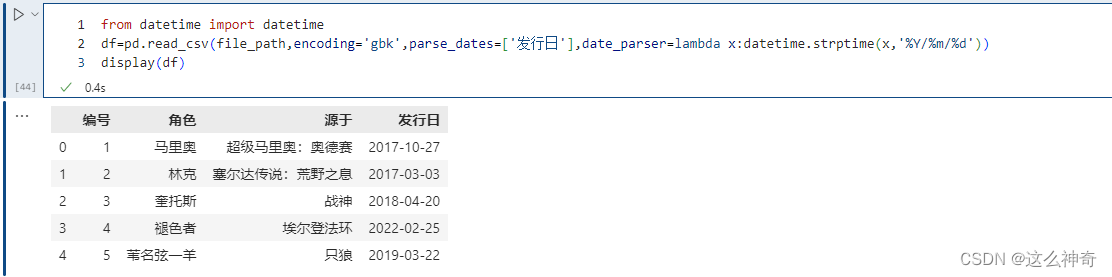## 22. infer_datetime_format

infer_datetime_format 参数默认为 False。如果设定为 True 并且 parse_dates 可用，那么 pandas 将尝试转换为日期类型，如果可以转换，转换方法并解析，在某些情况下会快 5~10 倍。

## 23. iterator

``````df=pd.read_csv(file_path,encoding='gbk',iterator=True)
display(df.get_chunk(2))

"""
编号	角色	源于	发行日
0	1	马里奥	超级马里奥：奥德赛	2017/10/27
1	2	林克	塞尔达传说：荒野之息	2017/3/3
"""

print(chunk.get_chunk(1))
"""

2	3	奎托斯	战神	2018/4/20
"""

# 文件还剩下三行，但是我们指定读取10，那么也不会报错，不够指定的行数，那么有多少返回多少
print(chunk.get_chunk(10))
"""

3	4	褪色者	埃尔登法环	2022/2/25
4	5	苇名弦一羊	只狼	2019/3/22
"""

try:
# 但是在读取完毕之后，再读的话就会报错了
chunk.get_chunk(5)
except StopIteration as e:
print("读取完毕")
# 读取完毕``````

## 24. chunksize

chunksize 整型，默认为 None，设置文件块的大小。

``````chunk = pd.read_csv(file_path, sep="\t", chunksize=2)
# 还是返回一个类似于迭代器的对象
# 调用get_chunk，如果不指定行数，那么就是默认的chunksize
print(chunk.get_chunk())
"""

0	1	马里奥	超级马里奥：奥德赛	2017/10/27
1	2	林克	塞尔达传说：荒野之息	2017/3/3
"""

# 但也可以指定
print(chunk.get_chunk(100))
"""

2	3	奎托斯	战神	2018/4/20
3	4	褪色者	埃尔登法环	2022/2/25
4	5	苇名弦一羊	只狼	2019/3/22
"""

try:
chunk.get_chunk(5)
except StopIteration as e:
print("读取完毕")
# 读取完毕``````

## 25. compression

compression 参数取值为 {‘infer’, ‘gzip’, ‘bz2’, ‘zip’, ‘xz’, None}，默认 ‘infer’，这个参数直接支持我们使用磁盘上的压缩文件。

``````# 直接将上面的.csv添加到压缩文件，打包成game_data.zip

## 27. encoding

encoding 指定字符集类型，通常指定为 ‘utf-8’。根据情况也可能是’ISO-8859-1’，本文中所有的`encoding='gbk'` ，主要原因为：我的数据是用Excel 保存成.CSV的，默认的编码格式为GBK；

``pd.read_csv(file_path,encoding='gbk',error_bad_lines=False, warn_bad_lines=True)``